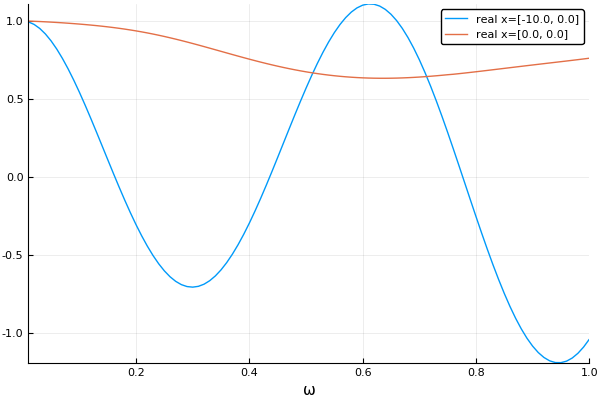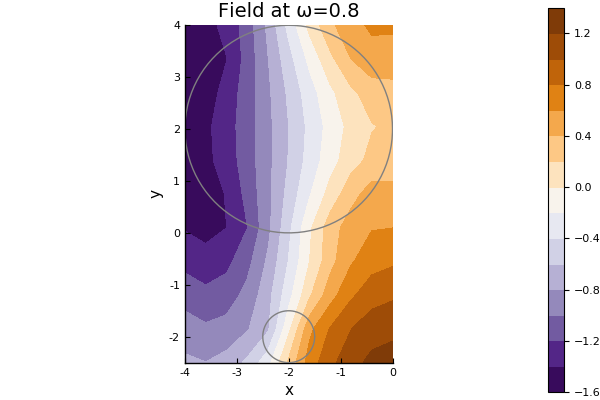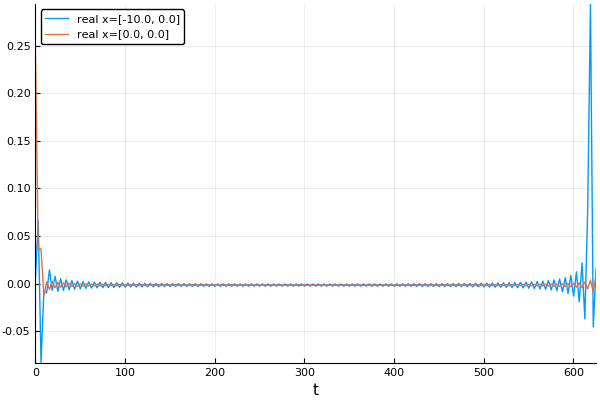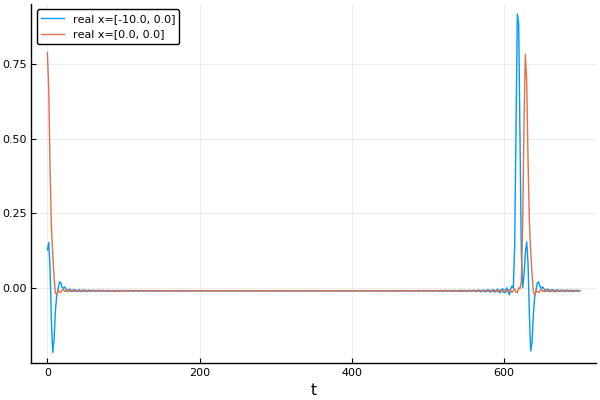16

8

4

2

# MultipleScattering

A Julia library for simulating, processing, and plotting multiple scattering of waves.

The library focuses on multipole methods (addition translation theorems) to solve the inhomogeneous Helmholtz equation (time-harmonic waves). Multipole methods are particularly efficient at solving scattering from particles in an infinite domain. This library is configured to use T-matrices (also known as scattering matrices) to represent scattering from particles with any shape and properties (currently implemented for acoustics). The package is setup to deal with different spatial dimensions and types of waves which satisfy Helmholtz equation's, e.g. acoustics, electromagnetism, elasticity. For details on some of the maths see Martin (1995) and Gower et al. (2017).

## Installation

This package is tested and works for Julia 0.6. To get started, download and include the library

``````Pkg.clone("https://github.com/JuliaWaveScattering/MultipleScattering.jl.git")
using MultipleScattering
``````

## Documentation

• masterdocumentation of the in-development version.

## Simple example

Define the properties of your host medium, for example

``````host_medium = Acoustic(2; ρ=1.0, c=1.0); # 2D acoustic medium with density ρ = 1.0 and soundspeed c = 1.0
``````

an acoustic medium in 2D with density 1 and wavespeed 1.

Next, define two dense, circular acoustic particles, the first centred at [-2,2] with radius 2 and the second at [-2,-2] with radius 0.5,

``````particle_medium =  Acoustic(2; ρ=10.0, c=2.0); # 2D acoustic particle with density ρ = 10.0 and soundspeed c = 2.0
p1 = Particle(particle_medium, Circle([-2.0,2.0], 2.0));
p2 = Particle(particle_medium, Circle([-2.0,-2.0], 0.5));
particles = [p1,p2];
``````

Lastly we define the source, for example an incident plane wave () using a helper function.

``````source = plane_source(host_medium; direction = [1.0,0.0])
``````

Once we have these three components, we can build our `FrequencySimulation` object

``````simulation = FrequencySimulation(particles, source)
``````

To get numerical results, we run our simulation for specific positions and angular frequencies,

``````x = [[-10.0,0.0], [0.0,0.0]]
max_ω = 1.0
ω = 0.01:0.01:max_ω
result = run(simulation, x, ω)
``````

### Plot

The package also provides recipes to be used with the `Plots` package for plotting simulations after they have been run. In our above simulation we ran the simulation for 100 different wavenumbers, and measured the response at the location (-10,0). We can plot the time-harmonic response across these wavenumbers by typing:

``````using Plots
plot(result)
``````For a better overview you can plot the whole field in space for a specific angular frequency by typing:

``````ω = 0.8
plot(simulation,ω)
``````This measures the field at lots of points in the domain, so we can get an understanding of what is happening for one particular angular frequency.

Note: most things in the package can be plotted by typing `plot(thing)` if you need an insight into a specific part of your simulation.

To calculate an incident plane wave pulse in time use:

``````time_result = frequency_to_time(result)
plot(time_result)
``````Or for a Gaussian impulse in time:

``````t_vec = LinRange(0.,700.,400)
time_result = frequency_to_time(result; t_vec = t_vec, impulse = GaussianImpulse(max_ω))
plot(time_result)
``````## More examples

There are a lot of defaults implicit in this basic example. Almost every part of the problem can be controlled, for example we can manually construct the set of particles, define their positions, radii and give them specific material properties. For all examples see here.

## Acknowledgements and contributing

This library was restructured from one written by Artur L Gower and Jonathan Deakin. Please contribute, if nothing else, criticism is welcome. We are relatively new to Julia, and this is our first package, if anything is untoward or even non-standard, please let us know.

07/10/2017

10 days ago

346 commits